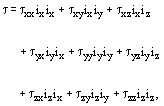# Normal Stress

In a rectangular or Cartesian coordinate system (x,y,z), the stress tensor τ is given by:
(1)where ix, iy and iz denote unit vectors in the x-direction, y-direction and z-direction, respectively. The components of τ may be interpreted thus:
• τxx is the x-component of force per unit area exerted across a plane surface element normal to the x-direction;
• τxy is the y-component of force per unit area exerted across a plane surface element normal to the x-direction; and so on. (Precisely analogous interpretations can be given to components of stress in any orthogonal coordinate system.)
Each component with identical suffices (τxx, τyy and τZZ) is called a normal stress since it represents a force in a direction per unit area normal to that direction. Each other component with different suffices (τxy, τyx, τxz, τzx, τyz and τzy) is called a Shear Stress.